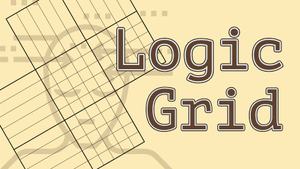### How to play

• The variables represents unique integers ranging from 1 to the number of variables;
• Multiplication is implicit: `AB` means `A` times `B`, or `A * B`;
• Based on the clues (equations and inequations), use the grid to create relations between variables and values:
• Click once on a square to mark that value as false;
• Click twice to assign the chosen value to the variable;
• Click three times to clear the square.
• The color of a clue changes after you assign values to all of its variables:
• GREEN means that the statement is true;
• RED means that the statement is false.
• Click on a clue to mark it as used;
• The game ends when all values are correctly assigned to the variables.

### Tips

• Figuring out which variables can't have the lowest or the highest values is a good starting point, e.g, `A > B` shows that `A ≠ 1`;
• Analyzing the hypothetical equation `C + D = 4`, we can make the following statements:
• `C = 1` and `D = 3` OR `C = 3` and `D = 1`;
• No other variable can be 1 or 3 (see Naked Pairs in our Sudoku Guide).
• Another quick tip: `A = 2B` means that `A` is even;
• Don't be afraid of using pen and paper. Some puzzles might require it.

• E = C + 6
• G = D + 2
• F = A + 2
• G = B + 4

• G = E + 1
• D = C + 1
• A = B + 6
• C = G + 1
• E = F + 1

• B = 4D
• A = 3C
• 4E = 3B
• G ≠ 5

### More games##### Logic Grid Puzzles

Complete the grid by using logic and the given clues of each problem.##### Greek Logic

Fill the grid using the Greek letters.##### IQ Tests